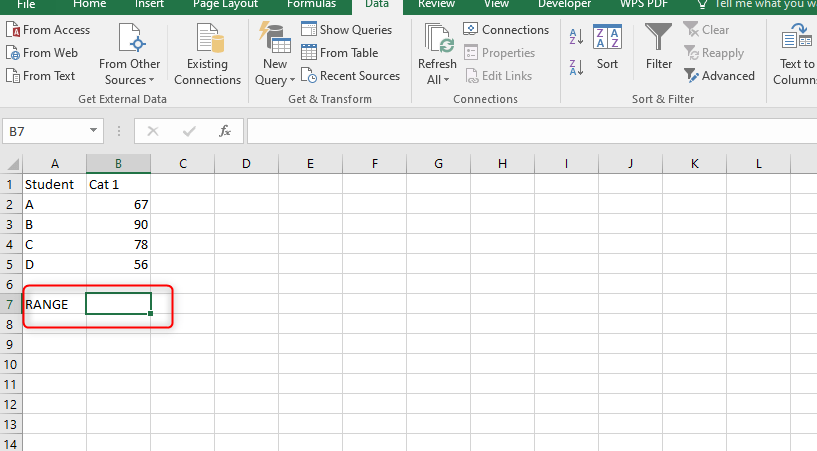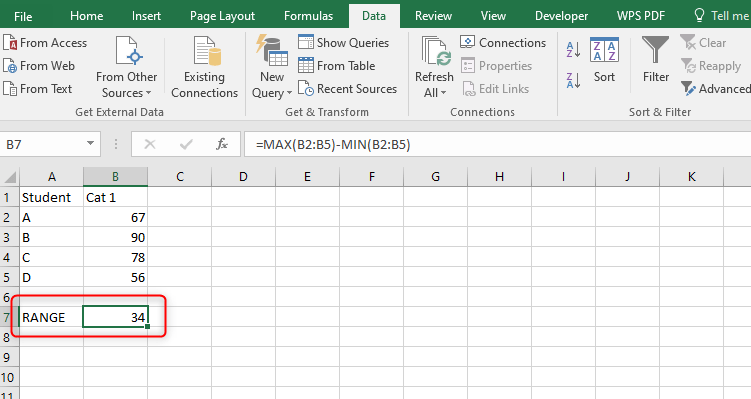# How to find Range in Excel

The range is a common term used in mathematics. It refers to the measurement between the maximum and minimum data of a given dataset. There are a million ways that are used to calculate the range of any dataset. Excel being a powerful statistical tool, can be used to calculate problems related to range.

Below are some methods in Excel that can be used to calculate the range of any dataset.

## Using the Max and Min Function

To find the range of any dataset, three operations are involved;

1. Finding the largest value

2. Smallest value

3. Finding the difference between the largest and smallest value.

Therefore, the Min and Max functions can be used to calculate the range of any dataset in Excel.

Steps;

1. Open a new or an existing worksheet. If you're working on a new worksheet, enter your dataset on the empty cells.2. Select another cell and name it "Range."3. On the selected cell, type the Min and Max function. Within the bracket of each function, enter the cells that contain your dataset. For example, B2:B10- to mean from cells 2 to cell 10 in column B.

=MAX(B2:B5)-MIN(B2:B5)4. Then, hit the Enter button. The formula will take both the largest and smallest value from your dataset, and then subtract the smallest from the largest value.## Using the SMALL and LARGE functions

Another way to find the range of a given dataset is to use the combination of SMALL and LARGE functions. Let us dive deeper into how to use these functions.

Steps;

1. Open a new or an existing worksheet. If you're working on a new worksheet, enter your dataset on the empty cells.2. Select another cell and name it "Range."3. The SMALL and LARGE functions take two parameters. That is the array part and the nth part. The nth part shows the value to be returned. For example, if you want to return the largest or smallest number in the dataset, the nth value will be 1.

4. On the selected cell, type the SMALL and LARGE functions.

=LARGE(B2:B5,1)-SMALL(B2:B5,1)5. Then, hit the Enter button and you will have the range value on the selected cell.## Using the Autosum feature in Excel

Here is another method to calculate the range in excel.

Steps;

1. Open a new or an existing worksheet. If you're working on a new worksheet, enter your dataset on the empty cells.

2. From the main screen, click on the "Formulas tab."

3. Then locate the "Autosum" within the "Function Library" section and click on its drop-down button.4. From the drop-down menu, there's a Min and Max button. Firstly, click on the Max button to calculate the maximum number within your dataset. Store it in one of the empty cells within the worksheet.5. Secondly, click on the Min button to get the smallest number in your data set.6. Manually, subtract the value in the Min cell from that in the Max cell.7. Finally, hit the Enter button.